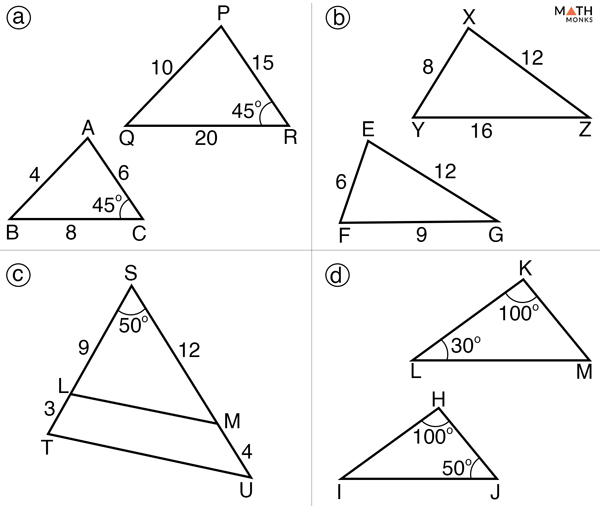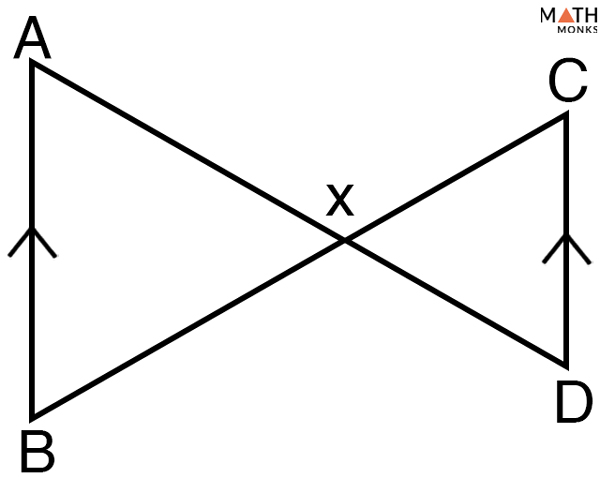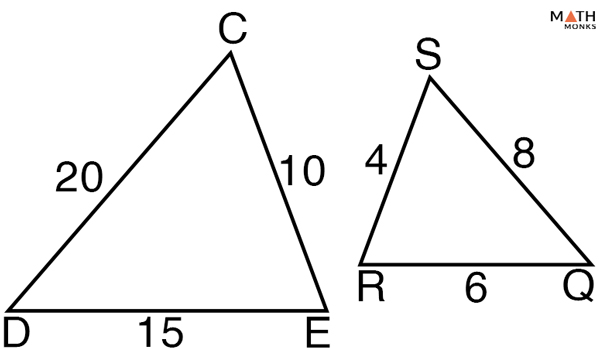# Similar Triangles

## What are Similar Triangles

Two triangles are similar if they have the same shape but are of different sizes. Thus mathematically, if two triangles are similar, then their corresponding sides are proportional and their corresponding angles are congruent. For example, all equilateral triangles are always similar.

Shown below are two triangles, ΔABC and ΔPQR that are similar, which is mathematically represented by the symbol ~. Here, ΔABC ~ ΔPQR.

Also, turning or flipping a triangle, and renaming it will form similar triangles.

## Properties

1. Has the same shape but size may vary; in ΔZYX and ΔPQR, ΔZYX > ΔPQR in size
2. Has each pair of corresponding angles congruent; here ∠Z ≅ ∠P, ∠Y ≅∠Q, and ∠X ≅ ∠R
3. Has the ratio of the corresponding sides same: here x/r = z/p = y/q

## Proving Triangles Similar: Rules

There are three ways to tell if triangles are similar, the conditions are given below:

### 1) Angle-Angle (AA) Rule

It states that if two angles in one triangle are equal to two angles of the other triangle, then the two triangles are similar.

From the above figure with AA rule, we can write

AB/EF = BC/FG = AC/EG and ∠B ≅ ∠F

Thus, to prove two triangles are similar, it is sufficient to show that two angles of one triangle are congruent to the two corresponding angles of the other triangle.

### 2) Side-Angle-Side (SAS) Rule

It states that if the ratio of their two corresponding sides is proportional and also, the angle formed by the two sides is equal, then the two triangles are similar.

From the above figure with SAS rule, we can write

AB/EF = BC/FG = AC/EG and ∠B ≅ ∠F, ∠C ≅ ∠G

Thus, to prove triangles similar by SAS, it is sufficient to show to sets of corresponding sides in proportion and the included angle to be congruent.

### 3) Side-Side-Side (SSS) Rule

It states that if all the three corresponding sides of one triangle are proportional to the three corresponding sides of the other triangle, then the two triangles are similar.

From the above figure with SSS rule, we can write

∠A ≅ ∠E ∠B ≅ ∠F, and ∠C ≅ ∠G

Thus, to prove triangles similar by SSS, it is sufficient to show that the three sets of corresponding sides are in proportion.

## How to Solve Problems on Similar Triangles

There are two types of similar triangle problems. One set requires proving whether a given set of triangles are similar and the other requires calculating the missing angles and the side lengths of similar triangles.

Let us solve some problems to understand the both the concept.Which of the given pairs of triangles are similar? Write the similarity rule that defines their relationship.

Solution:

a) ΔABC ~ ΔPQR by SSS similarity rule, b) Not similar, c) ΔSTU ~ ΔSVW by SAS similarity rule, d) ΔKLM ~ ΔHIJ by AA similarity ruleGiven below are two triangles. Are they similar?

Solution:

In ΔEFG, by angle sum property
∠E + ∠F + ∠G = 180°
80° + ∠F + 30° = 180°
∠F = 180° – (80° + 30°)
∠F = 70°
Similarly, in ΔLMN, by angle sum property
∠L + ∠M + ∠N = 180°
80° + 70° + ∠N = 180°
∠N = 180° – (80° + 70°)
∠N = 30°
Since,
∠F = ∠M = 70° and ∠N = ∠G = 30°
Thus, by AA rule,
ΔEFG ~ ΔLMNIn the given triangle, ΔABC with sides AE = 2cm, EB = 4 cm, and BC = 9 cm. Also EF || BC. Calculate EF.

Solution:

In ΔABC and ΔAEF,
∠EAF is common and ∠AEF = ∠ABC (corresponding angles)
Thus,
ΔABC ~ ΔAEF (AA rule)
Now, we can write
AE/AB = EF/BC
AE/AE + EB = EF/BC, here AE = 2cm, EB = 4 cm, BC = 9 cm
2/(2 + 4) = EF/9
EF = 3 cmIdentify the similar triangles and write the similarity statement that describes their relationship.

Solution:

In ΔXAB and ΔXDC,
∠ABX ≅ ∠DCX (Alternate Interior Angles)
∠AXB ≅ ∠DXC (Vertically opposite angles)
Thus, by AA rule,
ΔXAB ~ ΔXDCFind the value of x in the given pair of similar triangles.

Solution:

The given pair of triangles are similar by AA rule
Thus, we can write
FG/QR = EF/PQ, here FG = x, QR = 15, EF = 4, PQ = 5
x/15 = 4/5
x = 12Determine whether the given pairs of triangles are similar.

Solution:

The given pairs of triangles are similar by the SSS rule.
For the triangles to be similar the ratio of their corresponding sides should be equal
If ΔCDE ~ ΔSRQ
SR/CE = RQ/DE = SQ/CD
Now,
SR/CE = 4/10 = 2/5
RQ/DE = 6/15 = 2/5
SQ/CD = 8/20 = 2/5
Hence,
ΔCDE ~ ΔSRQSolve for x in the given figure.

Solution:

In the given figure,
ΔPQT ≅ ΔRST (all right triangles are congruent)
Also, ∠S ≅ ∠S (Reflexive Property)
Thus, by AA rule, ΔPQT ~ ΔRST
Now, QT/ST = PQ/RS (Corresponding sides of similar triangles are proportional)
=> x/12 = 5/3
=> x = (12 x 5)/3 = 20

## Similar Triangles and Scale Factor

When two triangles are similar, the minimum ratio of any two corresponding sides is called the scale factor.

In ΔEFG and ΔPQR, the ratio of their corresponding side lengths is 2/1. It can thus be said that the scale factor of ΔEFG to ΔPQR is 2.

Let us take an example to understand the concept better.

### How to Find the Scale Factor of Two Similar TrianglesFind the scale factor in the given pair of similar triangles.

Solution:

As we know, the corresponding sides of similar triangles are proportional by SSS rule,
Thus
Scale factor = 5/15 = 1/3
= 4/12 = 1/3
= 3/9 = 1/3
Thus, the scale factor between the two given triangles is 1:3

## Real–Life Applications

Similar triangles can be found everywhere around us and even somewhere we are unable to notice. Their importance is of utmost where it is beyond our reach to physically measure the distances and heights with simple measuring instruments. The numerous applications are mainly in the field of engineering, architecture, and construction. They are summarized below:

• Working out the heights of tall objects such as trees, buildings, and towers which are too hard to climb and measure with a measuring tape.
• Analyzing shadows that helps to determine the actual height of objects.
• Determining the distances from sky to a particular point on ground in aerial photography.
• Analyzing the stability of bridges during construction and also to measure the scale size of rooms in buildings.

## FAQs

Q1. Are all equilateral triangles similar?

Ans. Yes, all equiangular triangles are similar.

Q2. Are all isosceles triangles similar?

Ans. No, all isosceles triangles are not similar.

Q3. Are all right triangles similar?

Ans. No, right triangles are not always similar.

Q4. Are congruent triangles similar?

Ans. Yes, congruent triangles are always similar.

Q5. Are all equiangular triangles similar?

Ans. Yes, all equiangular triangles are similar.